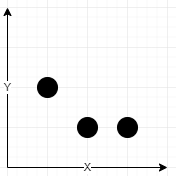Formatted question description: https://leetcode.ca/all/1620.html

# 1620. Coordinate With Maximum Network Quality (Medium)

You are given an array of network towers towers and an integer radius, where towers[i] = [xi, yi, qi] denotes the ith network tower with location (xi, yi) and quality factor qi. All the coordinates are integral coordinates on the X-Y plane, and the distance between two coordinates is the Euclidean distance.

The integer radius denotes the maximum distance in which the tower is reachable. The tower is reachable if the distance is less than or equal to radius. Outside that distance, the signal becomes garbled, and the tower is not reachable.

The signal quality of the ith tower at a coordinate (x, y) is calculated with the formula ⌊qi / (1 + d)⌋, where d is the distance between the tower and the coordinate. The network quality at a coordinate is the sum of the signal qualities from all the reachable towers.

Return the integral coordinate where the network quality is maximum. If there are multiple coordinates with the same network quality, return the lexicographically minimum coordinate.

Note:

• A coordinate (x1, y1) is lexicographically smaller than (x2, y2) if either x1 < x2 or x1 == x2 and y1 < y2.
• ⌊val⌋ is the greatest integer less than or equal to val (the floor function).

Example 1:Input: towers = [[1,2,5],[2,1,7],[3,1,9]], radius = 2
Output: [2,1]
Explanation:
At coordinate (2, 1) the total quality is 13
- Quality of 7 from (2, 1) results in ⌊7 / (1 + sqrt(0)⌋ = ⌊7⌋ = 7
- Quality of 5 from (1, 2) results in ⌊5 / (1 + sqrt(2)⌋ = ⌊2.07⌋ = 2
- Quality of 9 from (3, 1) results in ⌊9 / (1 + sqrt(1)⌋ = ⌊4.5⌋ = 4
No other coordinate has higher quality.

Example 2:

Input: towers = [[23,11,21]], radius = 9
Output: [23,11]


Example 3:

Input: towers = [[1,2,13],[2,1,7],[0,1,9]], radius = 2
Output: [1,2]


Example 4:

Input: towers = [[2,1,9],[0,1,9]], radius = 2
Output: [0,1]
Explanation: Both (0, 1) and (2, 1) are optimal in terms of quality but (0, 1) is lexicograpically minimal.


Constraints:

• 1 <= towers.length <= 50
• towers[i].length == 3
• 0 <= xi, yi, qi <= 50
• 1 <= radius <= 50

Related Topics:
Greedy

## Solution 1.

// OJ: https://leetcode.com/problems/coordinate-with-maximum-network-quality/

// Time: O(MNT) where M and N are the range of x and y values, T is the count of towers.
// Space: O(1)
class Solution {
public:
vector<int> bestCoordinate(vector<vector<int>>& A, int radius) {
int minX = INT_MAX, maxX = 0, minY = INT_MAX, maxY = 0, maxSum = 0;
vector<int> ans;
for (auto &t : A) {
int x = t, y = t;
minX = min(x, minX);
maxX = max(x, maxX);
minY = min(y, minY);
maxY = max(y, maxY);
}
for (int x = minX; x <= maxX; ++x) {
for (int y = minY; y <= maxY; ++y) {
int sum = 0;
for (auto &t : A) {
int a = t, b = t, q = t;
double d = sqrt(pow(x - a, 2) + pow(y - b, 2));
if (d <= radius) sum += (int)((double)q / (1 + d));
}
if (sum > maxSum) {
ans = {x, y};
maxSum = sum;
}
}
}
return ans;
}
};


Java

class Solution {
public int[] bestCoordinate(int[][] towers, int radius) {
int[] bestCoordinate = {0, 0};
int maxQuality = 0;
for (int x = 0; x <= 50; x++) {
for (int y = 0; y <= 50; y++) {
int[] coordinate = {x, y};
int quality = 0;
for (int[] tower : towers) {
double distance = getDistance(coordinate, tower);
quality += (int) Math.floor(tower / (1 + distance));
}
if (quality > maxQuality) {
bestCoordinate = coordinate;
maxQuality = quality;
}
}
}
return bestCoordinate;
}

public double getDistance(int[] coordinate, int[] tower) {
return Math.sqrt((tower - coordinate) * (tower - coordinate) + (tower - coordinate) * (tower - coordinate));
}
}